﻿ 考虑平衡负荷的混合遗传模拟退火柔性车间调度的研究 Research on Flexible Genetic Shop Scheduling with Mixed Genetic Simulated Annealing Considering Balanced Load

Computer Science and Application
Vol. 09  No. 07 ( 2019 ), Article ID: 31447 , 10 pages
10.12677/CSA.2019.97159

Research on Flexible Genetic Shop Scheduling with Mixed Genetic Simulated Annealing Considering Balanced Load

Duo Shao

School of Computer Science and Technology, Tianjin Polytechnic University, Tianjin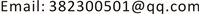Received: Jul. 6th, 2019; accepted: Jul. 19th, 2019; published: Jul. 26th, 2019ABSTRACT

In this paper, a hybrid genetic simulated annealing algorithm is proposed for flexible shop scheduling problem. The algorithm uses genetic algorithm to solve the problem of sorting and global search. It uses simulated annealing to perform local search. In the process of solving, the machine is allocated according to the load condition of the machine. The effectiveness of the algorithm is verified by an example test.

Keywords:Flexible Job-Shop Scheduling, GASA, Balanced Load1. 引言

2. 作业车间调度问题

2.1. 作业车间调度问题表述及其约束条件

FJSSP可以描述为：

1) 工件集 $J=\left\{{J}_{\text{1}},{J}_{2},\cdots ,{J}_{n}\right\}$ ，Ji为第i个工件， $\text{1}\le i\le n$

2) 机器集 $M=\left\{{M}_{1},{M}_{2},\cdots ,{M}_{m}\right\}$ ，Mj为第j号机器， $\text{1}\le j\le m$

3) 工序集 $O=\left\{{O}_{1},{O}_{2},\cdots ,{O}_{n}\right\}$ ，Oi为第i个工件的工序， $\text{1}\le i\le n$

4) 工序子集 ${O}_{i}=\left\{{O}_{i1},{O}_{i2},\cdots ,{O}_{im}\right\}$ ，Oik为第i个工件的第k道工序使用的机器号， $1\le k\le m$${O}_{ik}=1$ 表示工件Ji的第k道工序由机器号加工， $1\le k\le m$

5) 每道工序加工的使用时间 ${T}_{i}=\left\{{T}_{i1},{T}_{i2},\cdots ,{T}_{in}\right\}$ ，Tik为第i个工件的第k道工序所使用的机器号完成所消耗的时间， $\text{1}\le k\le n$

FJSSP需要满足以下的约束条件：

1) 每个加工机器彼此互相独立，不考虑机器故障带来的影响，并且所有的机器都是从(t = 0)时刻开始工作。

2) 所有的工件彼此互相独立，任一工件的加工不会影响其他工件的加工，所有工件均可在开始时刻加工。

3) 加工过程中不能突然改变工件工艺，所有的工件的工序在排产以前就已经确定。

4) 所有机器的加工每件的时间由机器本身的加工效率和工艺决定和确定，加工过程中不会对任何工件的任一工序的时间有所改变。

5) 每台设备每个时刻只能加工一个工件的一个工艺，同一工艺不能在多台机器上加工，一道工艺在某台加工设备上一旦开始加工，直至该工艺加工完成，中间不能中断。

6) 加工机器严格遵守工件工序的加工时间。

$C=\mathrm{min}\left(\underset{1\le j\le n}{\mathrm{max}}\left(\underset{1\le j\le m}{\mathrm{max}}{e}_{j}\right)\right)$ (1)

2.2. 平衡负荷的设计

${T}_{\text{total}}={\sum }_{j=1}^{m}{T}_{j}$ (2)

$\overline{T}=\frac{{T}_{\text{total}}}{M}$ (3)

$Nlb=\sqrt{{\sum }_{j=1}^{k}\left({T}_{j}+{T}_{ij}-\overline{T}\right)/M}$ (4)

3. 基于遗传和模拟退火的车间调度算法

3.1. 编码

3.2. 适应度函数设计

$F=\frac{1}{{P}_{n}}$ (5)

3.3. 选择操作

1) 首先确定种群在下一代中的生存数目：

${N}^{*}={\sum }_{i=1}^{N}⌊N\ast {F}_{i}/{\sum }_{i=1}^{N}{F}_{i}⌋$ ( $i=1,2,\cdots ,N$ ) (6)

N为种群的规模，Fi为第i个染色体的适应度值。

2) 根据二元锦标赛方式选择较好的个体，即每次从种群中随机选出两个个体进行对比，将适应度较高的个体保存到下一代中，重复这个过程直至下一代新种群的数量达到预期数量N*

${C}_{i}^{*}=\left\{\begin{array}{l}{C}_{i},{F}_{i}>{F}_{i+1}\\ {C}_{i+1},{F}_{i}<{F}_{i+1}\end{array}$ (7)

3.4. 交叉操作

1) 在种群中随机选取两个P1和P2染色体作为父代染色体，并初始化基因个数与父代个数相同的C1和C2，作为下一代个体。

2) 首先随机划分工件集 $\left\{\text{1},\text{2},\cdots ,n\right\}$ 为一个非空子集set1，set2$se{t}_{\text{1}}\cap se{t}_{\text{2}}=\varnothing$

3) 将P1中存在的与set1集合中相同的基因复制到C1中，复制到C1中的基因位置也与在P1位置对应相同。

4) 将P2中不存在于set1集合中的元素按顺序复制到C1的空缺位置中，如图1

${P}_{c}=\left\{\begin{array}{l}0.9-0.3\frac{{F}^{\prime }-{F}_{\text{avg}}}{{F}_{\mathrm{max}}-{F}_{\text{avg}}},{F}^{\prime }\ge {F}_{avg}\\ 0.9,{F}^{\prime }\le {F}_{\text{avg}}\end{array}$ (8)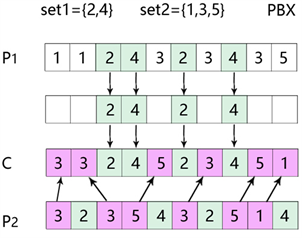Figure 1. PBX cross graph

3.5. 变异操作Figure 2. Gene variation graph

${P}_{m}=\left\{\begin{array}{l}0.9-0.3\frac{{F}^{\prime }-{F}_{\text{avg}}}{{F}_{\mathrm{max}}-{F}_{\text{avg}}}\\ 0.9,{F}^{\prime }\le {F}_{\text{avg}}\end{array},{F}^{\prime }\ge {F}_{avg}$ (9)

3.6. 模拟退火操作

1) 初始化：初始温度为T(充分大)，终止温度Tmin，初始化种群P，令当前状态为Pi，最优解 ${P}^{*}={P}_{i}$ ，t = 0，设置循环计数器k，降温系数d，L表示Metropolis算法第次迭代时产生的变换个数；

2) 产生新状态S'，计算增量 $\Delta {f}^{\prime }=f\left({{P}^{\prime }}_{i}\right)-f\left({P}_{i}\right)$

3) 判断ΔC'，若 $\Delta {C}^{\prime }>0$ ，以概率 $\mathrm{exp}\left(-\Delta {f}^{\prime }/t\right)$ 接受P'为当前的状态，P'被接受则令P' = P，令t = t + 1；否则接受P'为当前的状态，计算增量 $\Delta {C}^{\prime }=C\left({{P}^{\prime }}_{i}\right)-C\left({P}_{i}\right)$$\Delta {C}^{\prime }>0$${P}^{*}={P}^{\prime }$ ，令t = 0；

4) 判断是否 $t\ge {L}_{k}$ ，当前的状态是否无变化，如果是则结束循环；

5) 判断k是否终止，如果终止则令k = k + 1；转到步骤2，否则结束；

6) 重复以上操作直到对种群中的所有个体都完成了搜索。

3.7. 算法流程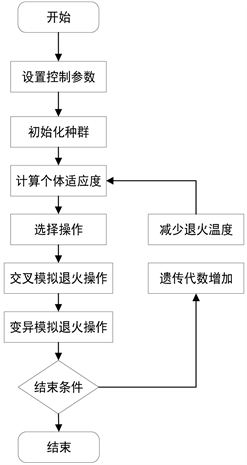Figure 3. Algorithm flow chart

4. 仿真比较及分析

4.1. 仿真试验1Table 1. Comparison table of the results of experiment 1

4.2. 仿真试验2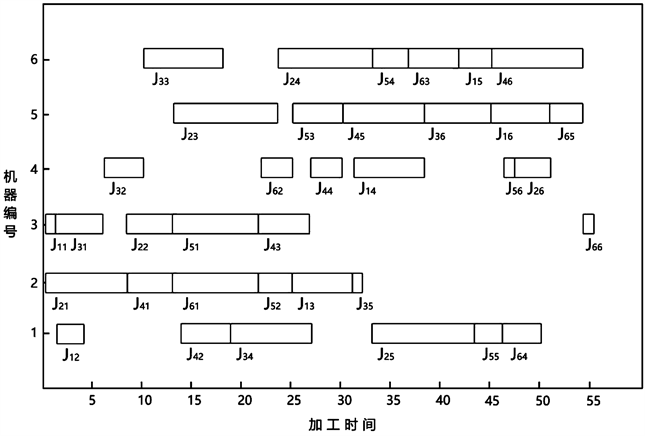Figure 4. Optimal scheduling diagram of FT06 example

Table 2. Job shop scheduling example table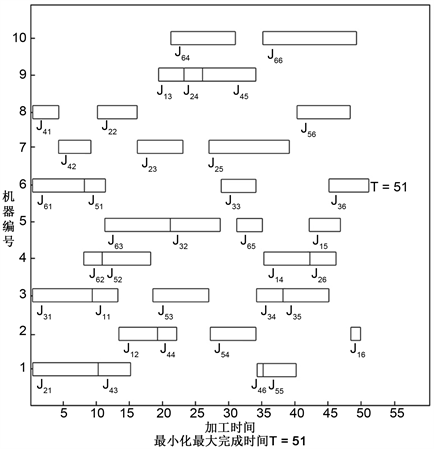Figure 5. The optimal scheduling diagram of job shop scheduling example in experiment 2Table 3. Test and comparison results of Job Shop scheduling example table

5. 结论

Research on Flexible Genetic Shop Scheduling with Mixed Genetic Simulated Annealing Considering Balanced Load[J]. 计算机科学与应用, 2019, 09(07): 1416-1425. https://doi.org/10.12677/CSA.2019.97159

1. 1. Bruker, P. and Schlie, R. (1990) Job Shop Scheduling with Multi-Purpose Machines. Computing, 45, 369-375. https://doi.org/10.1007/BF02238804

2. 2. 潘全科, 王文宏, 朱剑英, 等. 基于粒子群优化和变邻域搜索的混合调度算法[J]. 计算机集成制造系统, 2007, 13(2): 323-328.

3. 3. 何利, 刘永贤, 谢华龙, 刘笑天. 基于粒子群算法的车间调度与优化[J]. 东北大学学报(自然科学版), 2008, 29(4): 555-568.

4. 4. 黄歆雨. 基于混合遗传算法的柔性作业车间动态调度问题研究[D]: [硕士学位论文]. 福建: 福州大学, 2016.

5. 5. 周鑫, 马跃, 胡毅. 求解车间作业调度问题的混合遗传模拟退火算法[J]. 小型微型计算机系统, 2015, 27(6): 184-193.

6. 6. 梁旭, 黄明, 常征. 求解车间调度问题的一种新遗传退火混合策略[J]. 计算机集成制造系统, 2005, 2(36): 370-374.

7. 7. 刘巍巍, 马雪丽, 刘晓冰. 面向柔性作业车间调度问题的改进邻域搜索算法[J]. 计算机应用与软件, 2015, 32(4): 234-238.

8. 8. 黄学文, 张晓彤, 孙榕, 李冠雄. 基于遗传算法的工艺路径柔性调度算法[J]. 运筹与管理, 2018, 27(6): 184-193.

9. 9. 赵卫. 模拟退火遗传算法在车间作业调度中的应用[J]. 计算机仿真, 2011, 28(7): 361-364.

10. 10. 陈成, 邢立宁. 求解柔性作业车间调度问题的遗传——蚁群算法[J]. 计算机集成制造系统, 2011, 17(3): 615-621.

11. 11. Kacem, I., Hammadi, S. and Borne, P. (2002) Approach by Localization and Multi-Objective Evo-lutionary Optimization for Flexible Job-Shop Scheduling Problems. IEEE Transactions on Systems, Man, and Cybernet-ics, Part C (Applications and Reviews), 32, 1-13.

12. 12. 薛宏全, 魏生民, 张鹏, 杨琳. 基于多种群蚁群算法的柔性作业车间调度研究[J]. 计算机工程与应用, 2013, 49(24): 243-248, 261.